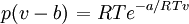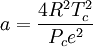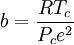# Dieterici equation of state$\left. p(v-b) \right.= RT e^{-a/RTv}$$a = \frac{4R^2T_c^2}{P_ce^2}$$b=\frac{RT_c}{P_ce^2}$